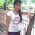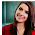# Computer System Architecture Set 3

## Questions 21 to 30

21.
A combinational circuit that converts binary information from n input lines to a maximum of  unique output lines is,
 (a) Decoder (b) Encoder (c) Full-adder (d) Full-subtractor (e) Half-subtractor.
22.
The correspondence between the main memory blocks and those in the cache is specified by
 (a) Mapping function (b) Replacement algorithm (c) Hit rate (d) Miss penalty (e) Segment function.
23.
The CPU nearly delays its operation for one memory cycle, to allow direct memory I/O transfer. This process is called,
 (a) Burst transfer (b) Cycle waiting (c) Cycle stealing (d) Cycle interrupting (e) Cycle execution.
24.
The control condition is terminated with
 (a) Comma (b) Semicolon (c) Colon (d) Hash (e) Dot.
25.
What are the missing values in the truth table of a half-adder given below?
x       y       C      S
0       0       --       --
0       1       0       1
1       0       0       1
1       1       1       0
 (a) x (b) y (c) 0 (d) 1 (e) Indeterminate.
26.
What are the building blocks of combinational circuits?
 (a) Flip-flops (b) Logical gates (c) Latches (d) Registers (e) Decoders.
27.
x + xy = x is called,
 (a) Commutative Law (b) Associative Law (c) Distributive Law (d) Absorption Law (e) Identity Law.
28.
What is BCO equivalent of 011111000?
 (a) 370 (b) 307 (c) 703 (d) 730 (e) None of the above.
29.
Boolean functions expressed as a --------- of minterms or ---------- of maxterms are said to be in a canonical form.
 (a) Product, Sum (b) Sum, Product (c) Subtract, Divide (d) Divide, Subtract (e) Product, Divide.
30.
Which of the following modes are used to handle data transfer to and from peripherals?
 (a) Programmed I/O (b) Interrupted-initiated I/O (c) Direct memory access (d) Programmed I/O, Interrupted-initiated I/O, Direct memory access (e) Programmed I/O, Direct memory access.

29  30  31  32  33  34  35  36  37  38  39  Next >>

1.2.3.